# Concise Selina Solutions for Class 9 Maths Chapter 19- Mean and median

Selina Solutions are considered to be very useful when you are preparing for the ICSE Class 9 maths exams. Here, we bring to you detailed answers and solutions to the exercises of Selina Solutions for Class 9 Maths Chapter 19- Mean and Median. These questions have been devised by the subject matter experts as per the syllabus prescribed by the CISCE for the ICSE.

Here we have provided the complete solutions of Class 9 Maths Chapter 19- Mean and Median in PDF format. Here, the PDF of the Class 9 Maths Chapter 19 Selina solutions is available which can be downloaded as well as viewed online. Students can also avail these Selina solutions and download it for free to practice them offline as well.

## Download PDF of Selina Solutions for Class 9 Maths Chapter 19:-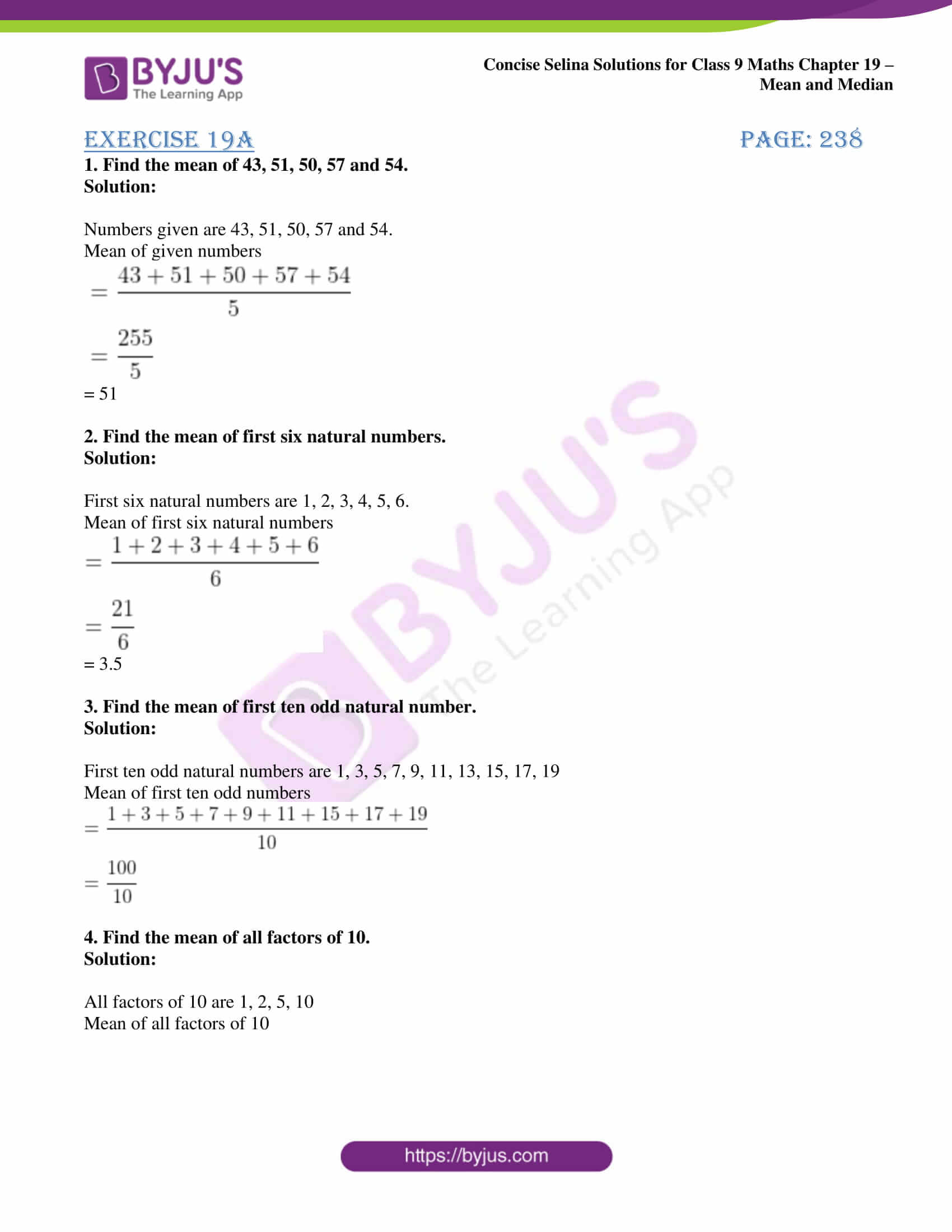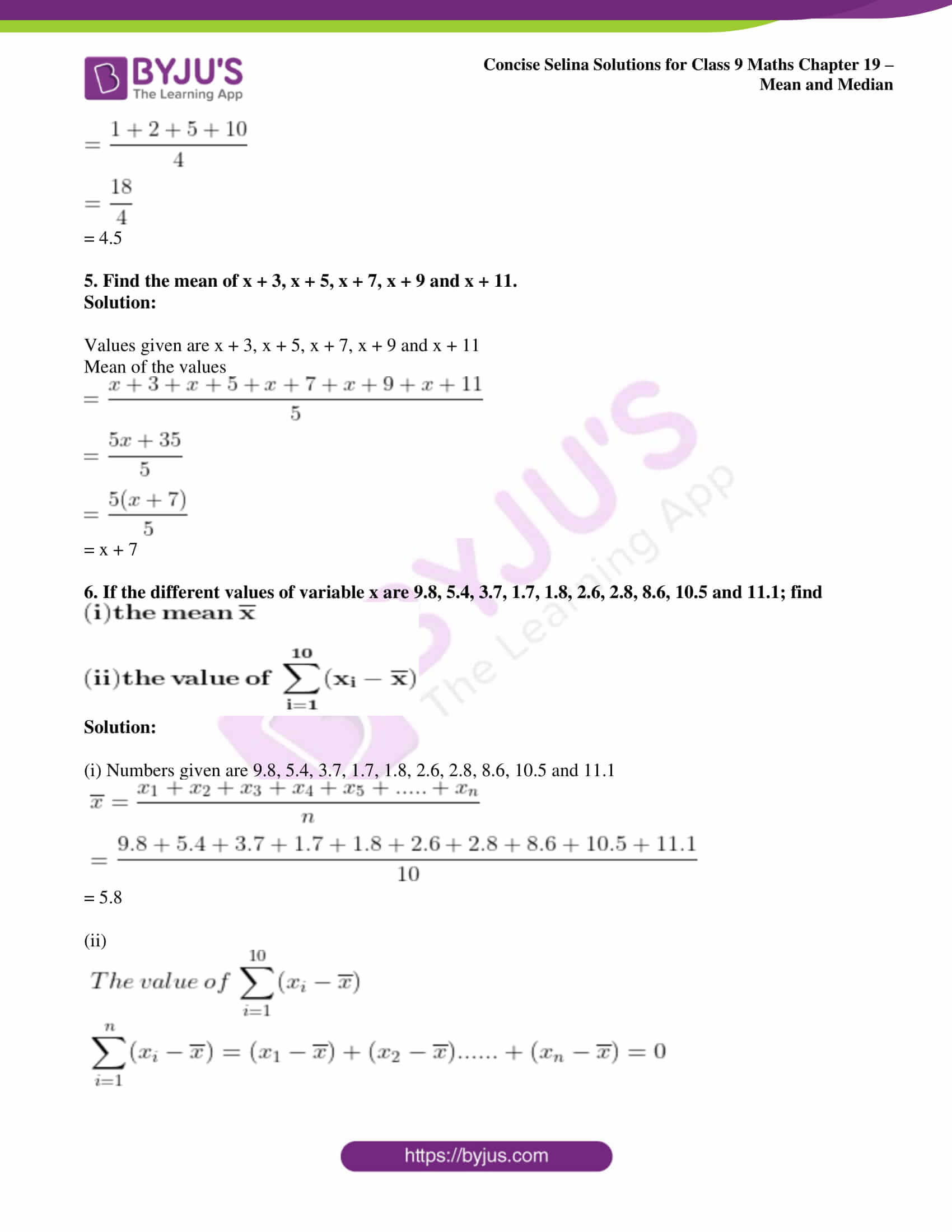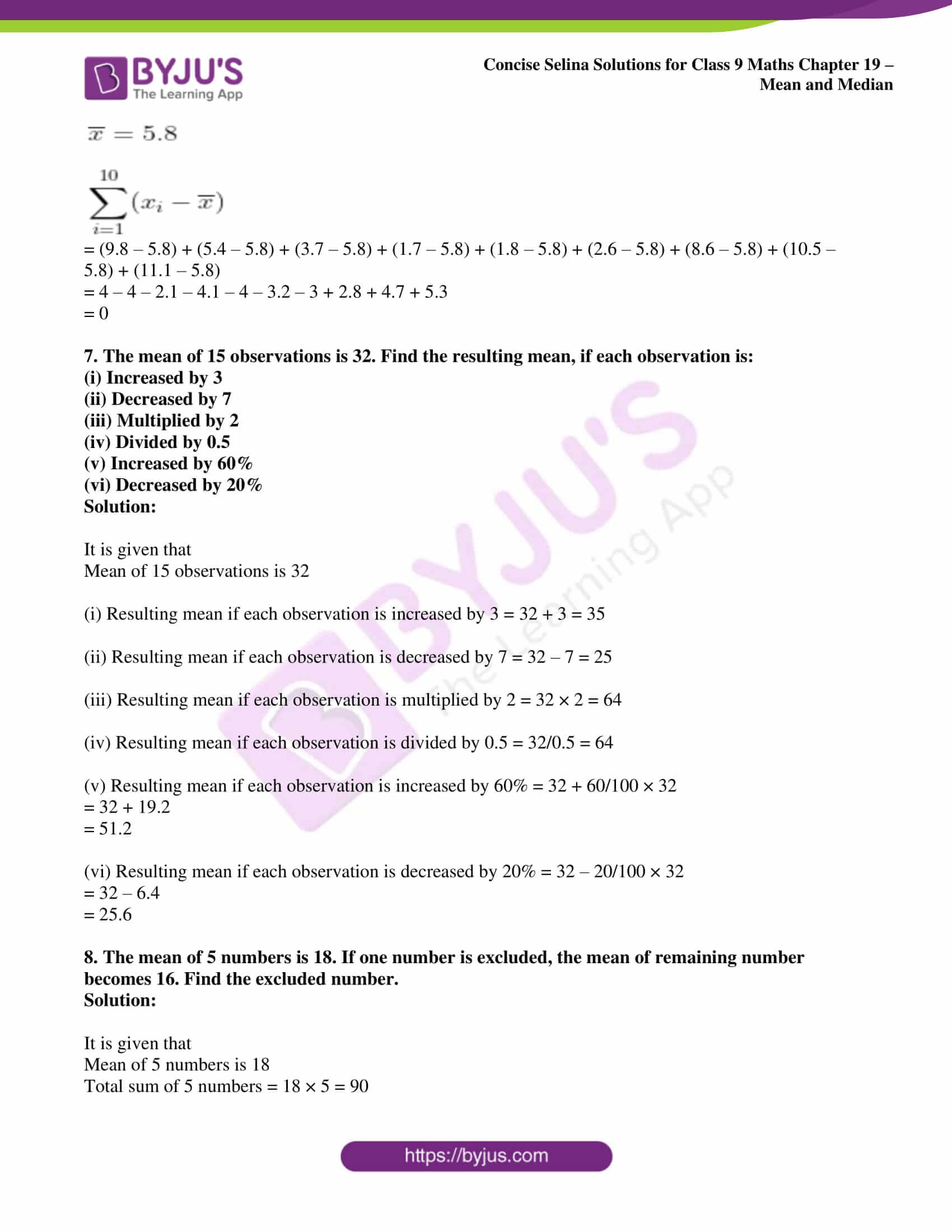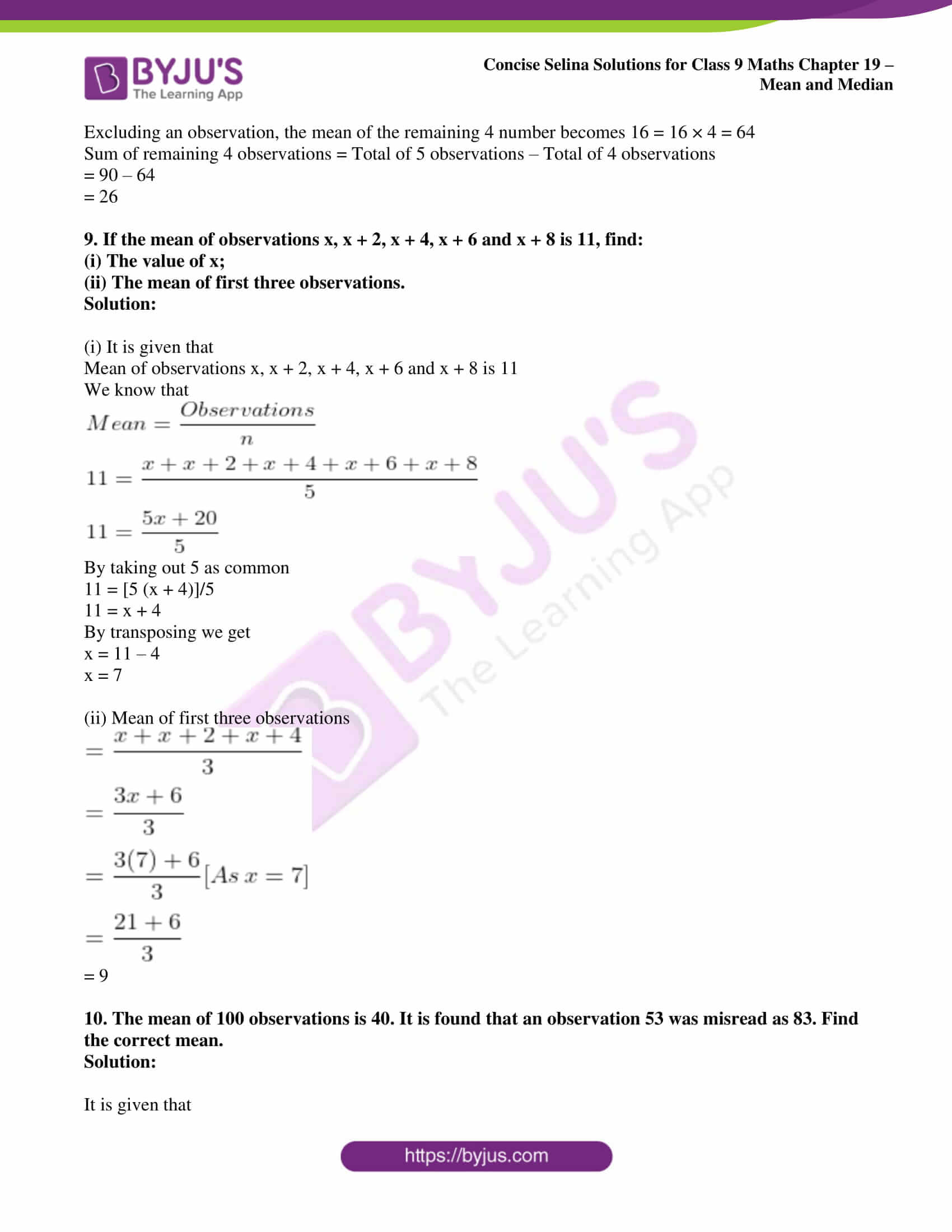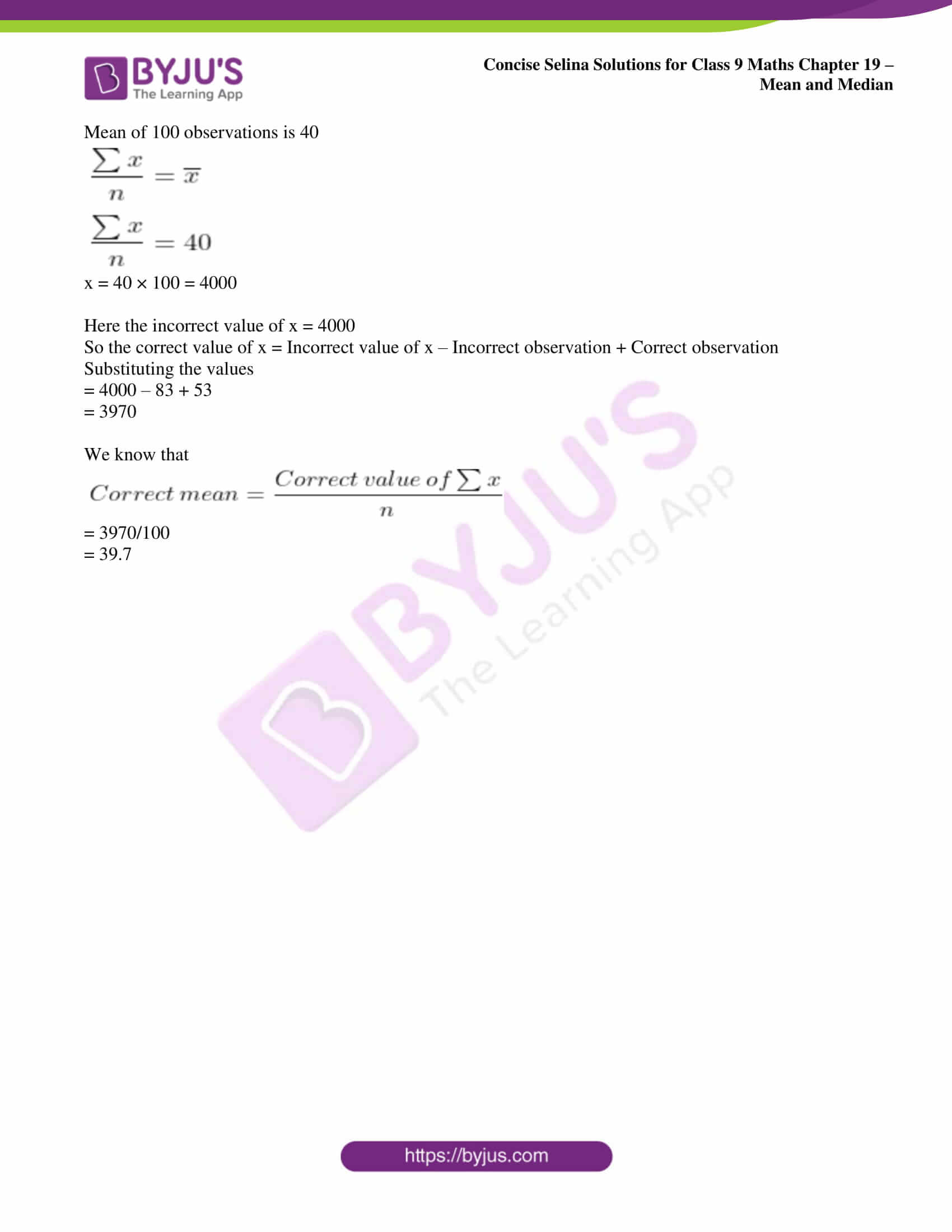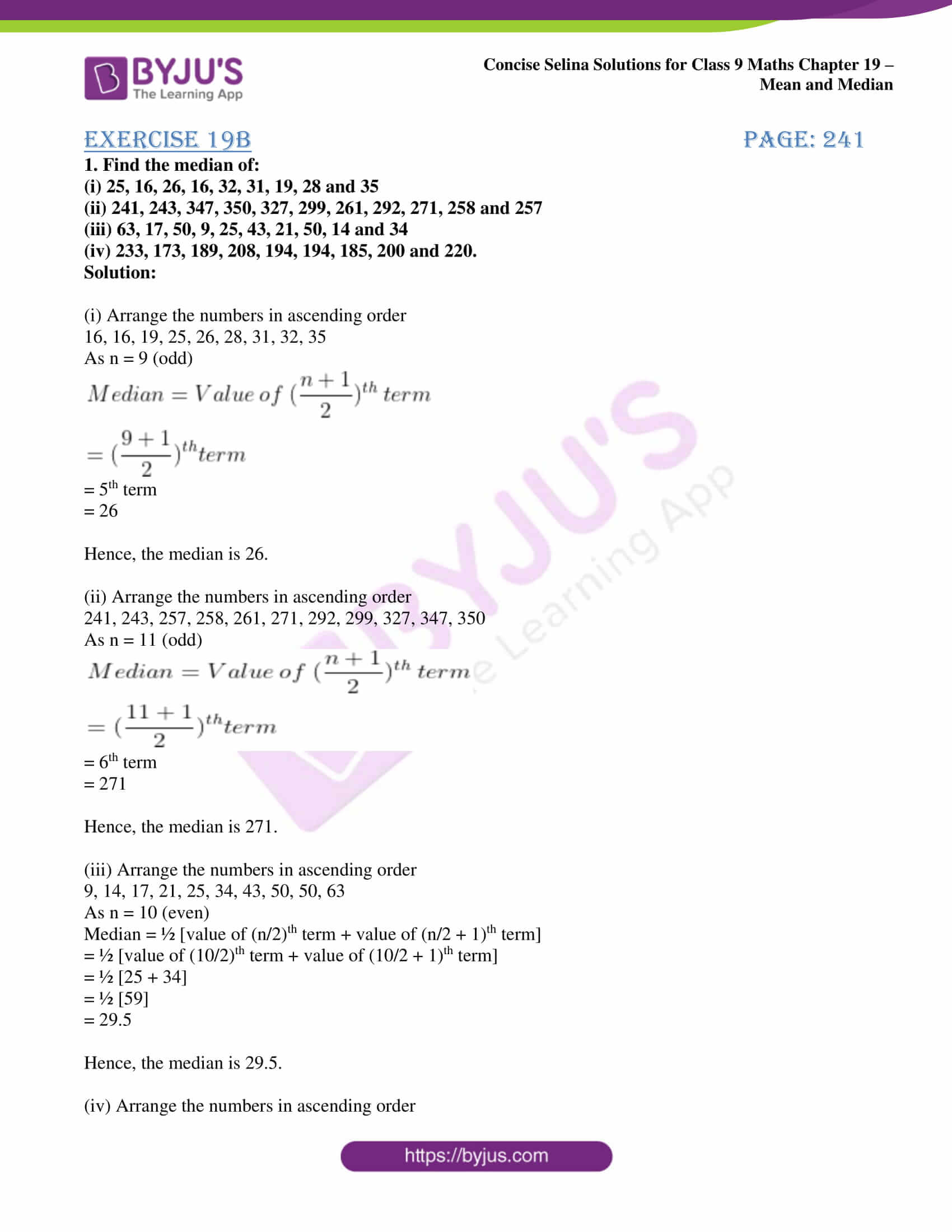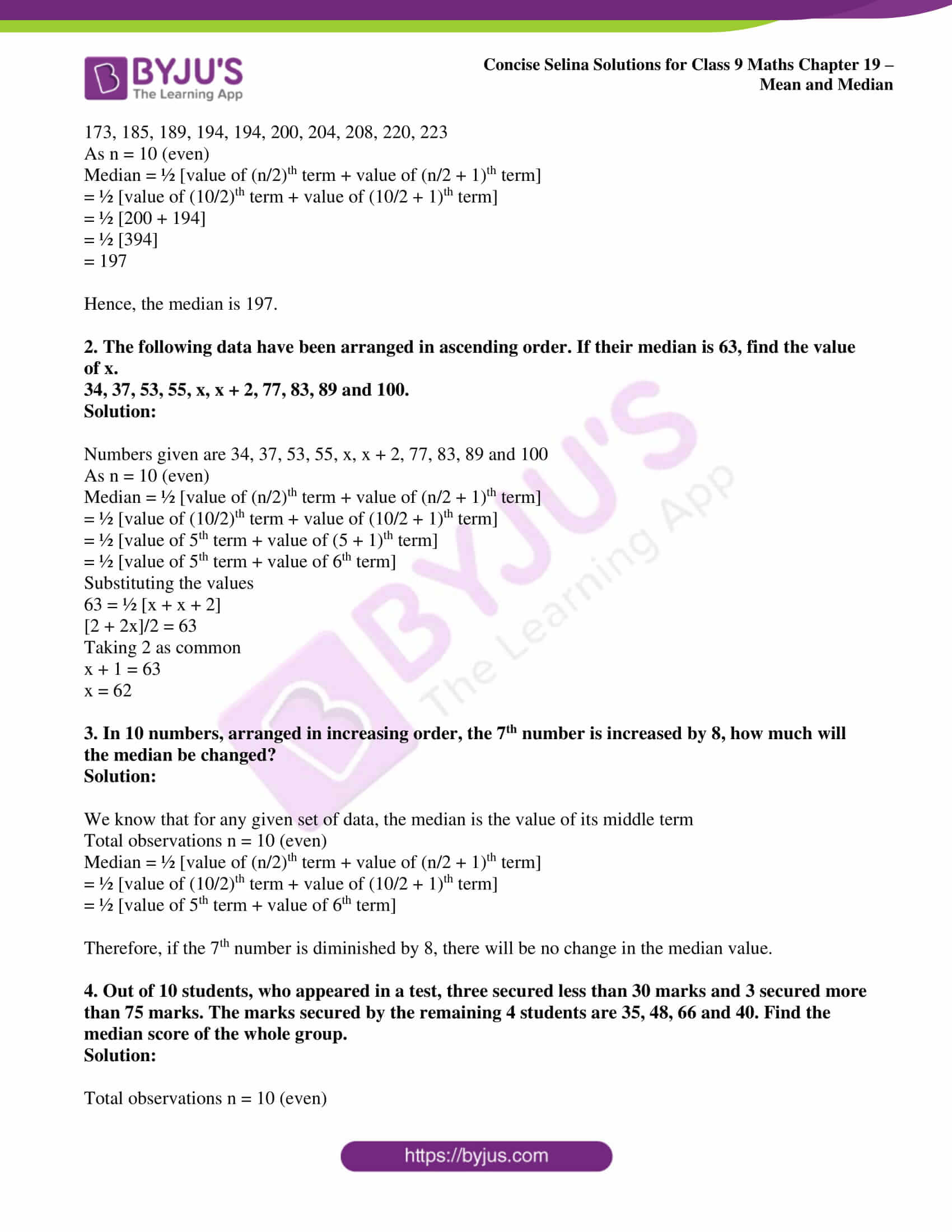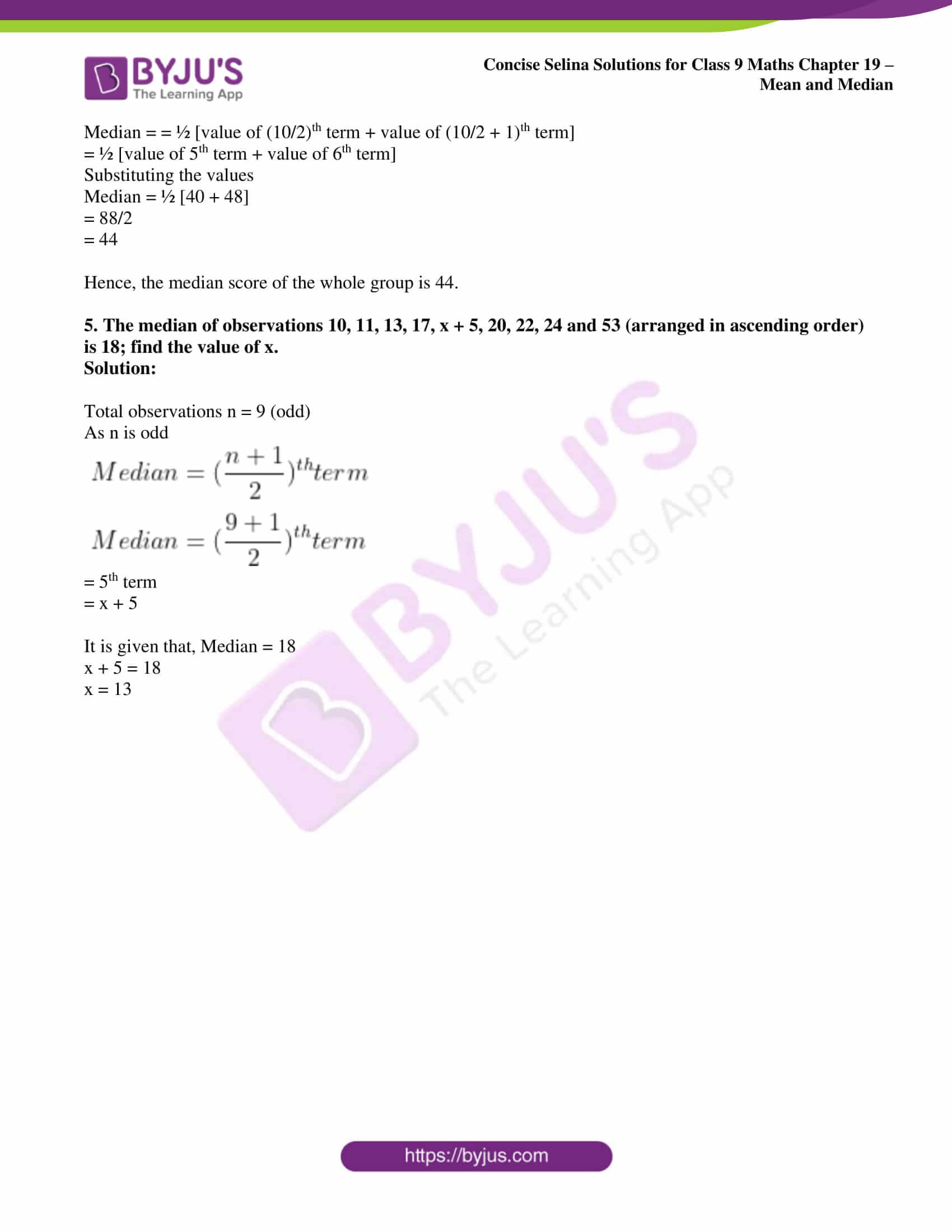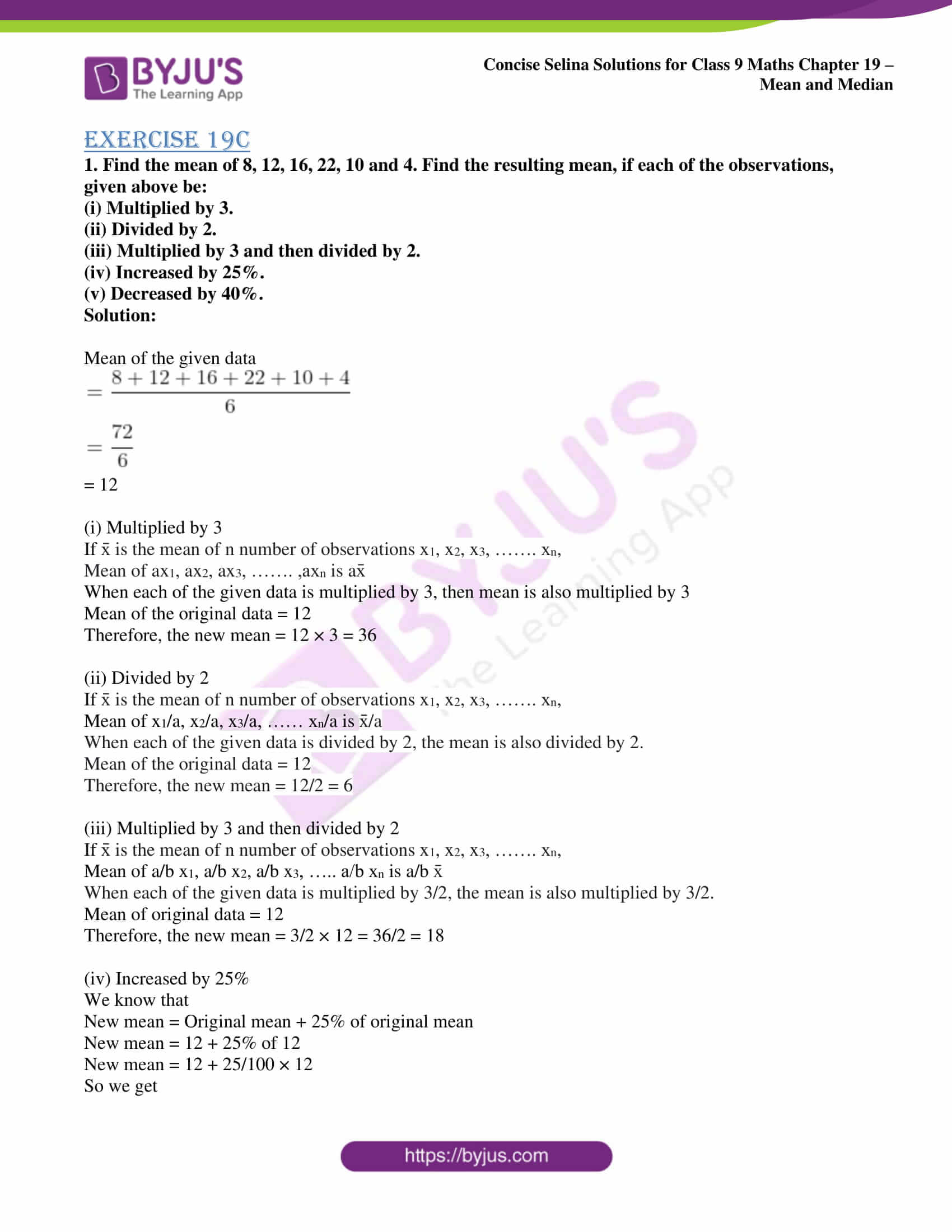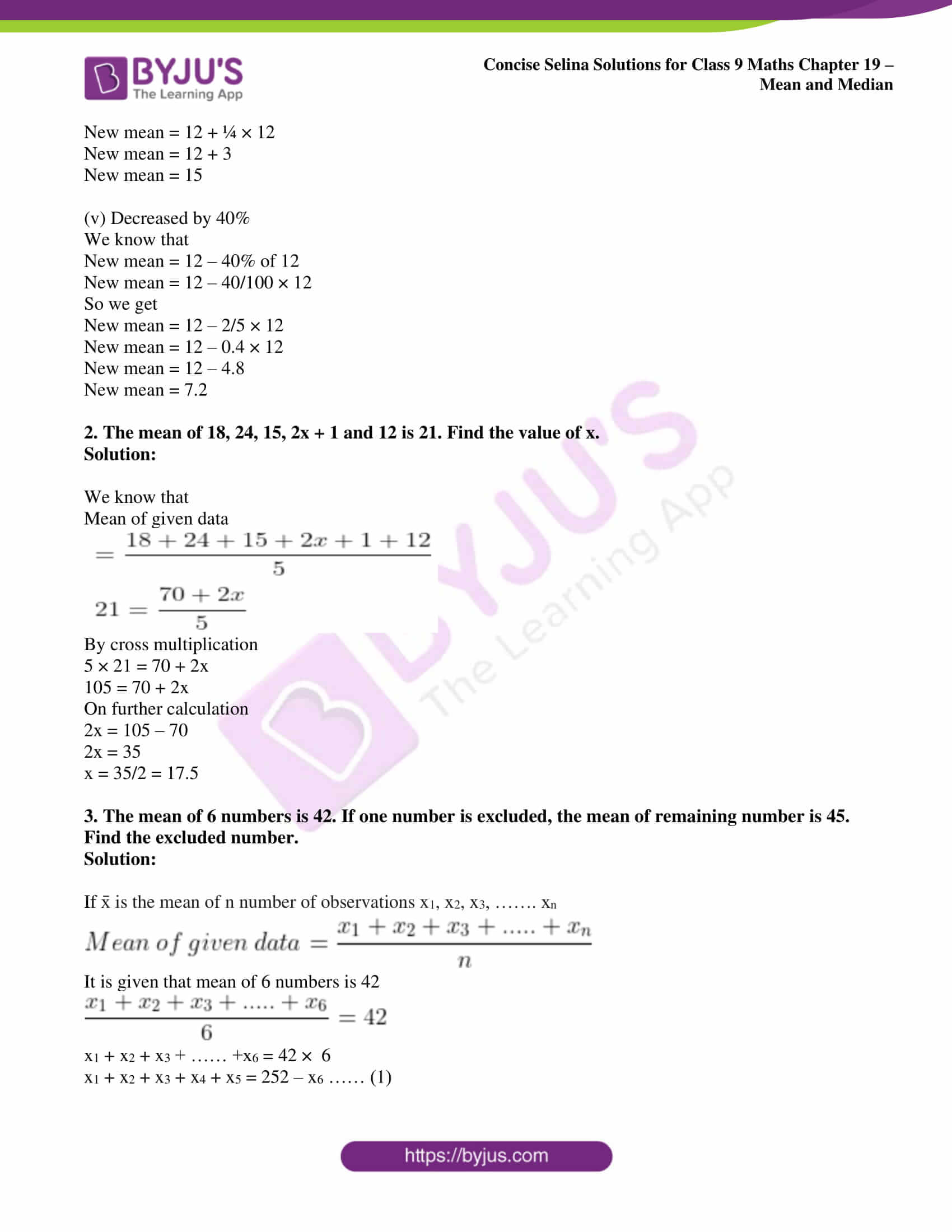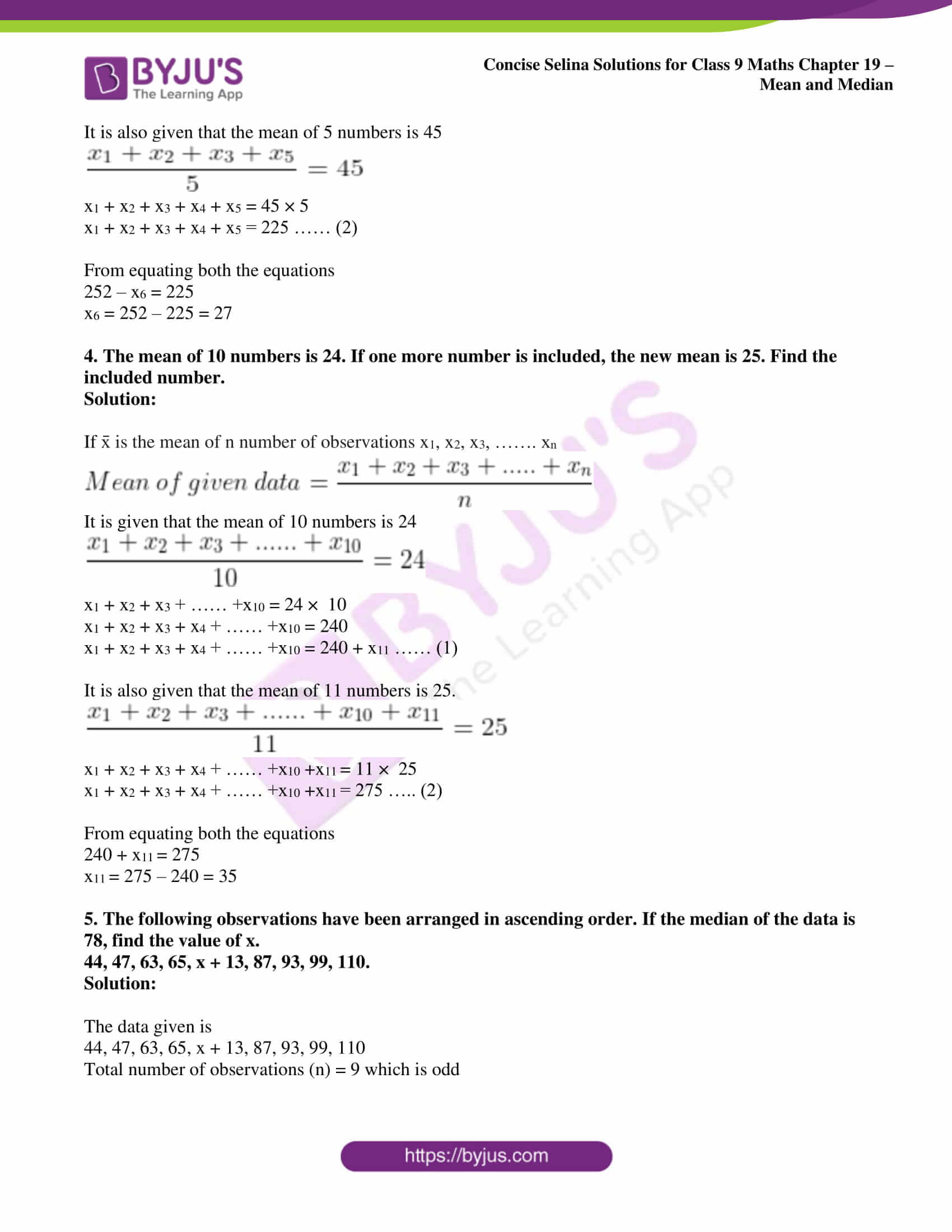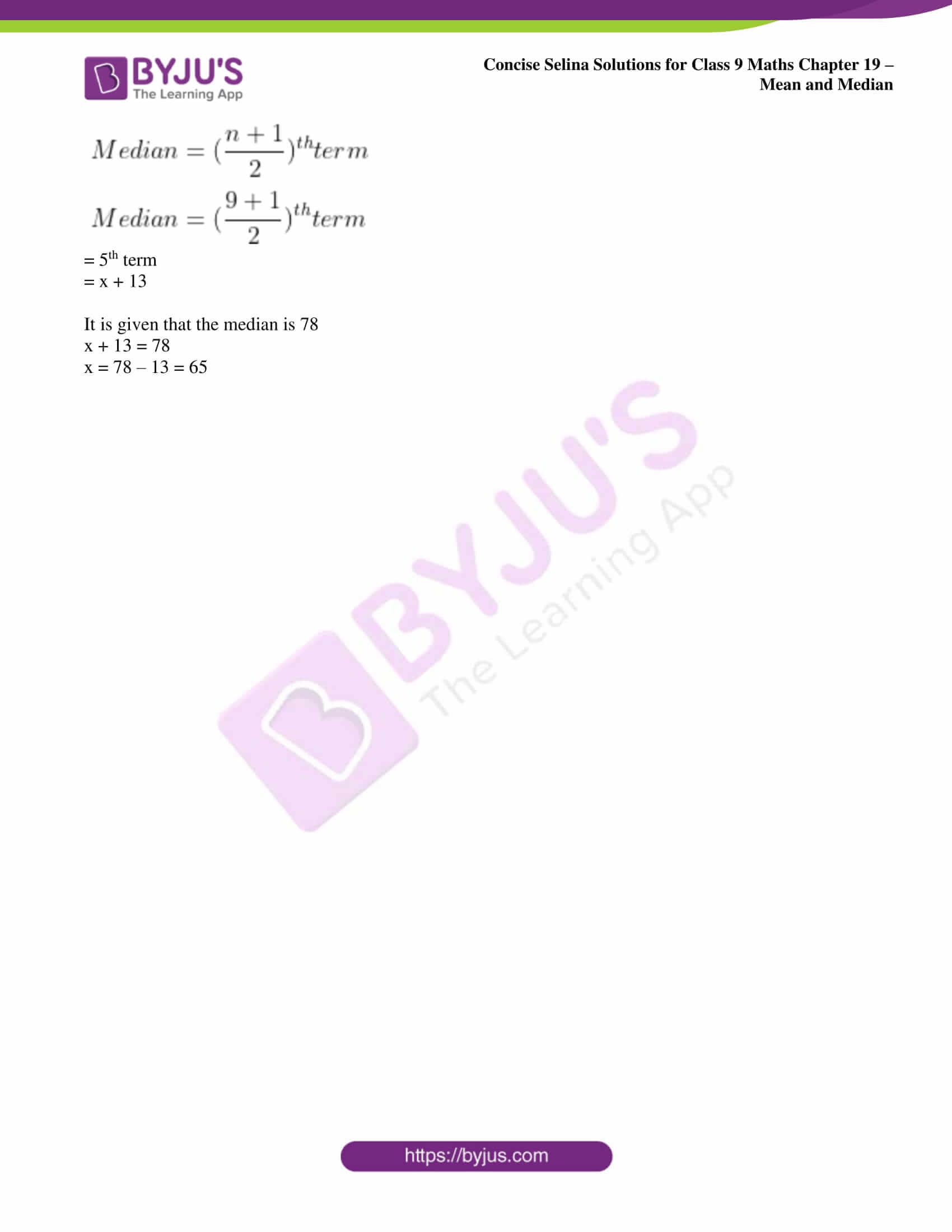Exercise 19A page: 238

1. Find the mean of 43, 51, 50, 57 and 54.

Solution:

Numbers given are 43, 51, 50, 57 and 54.

Mean of given numbers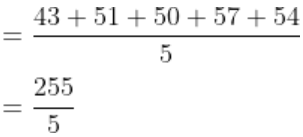= 51

2. Find the mean of first six natural numbers.

Solution:

First six natural numbers are 1, 2, 3, 4, 5, 6.

Mean of first six natural numbers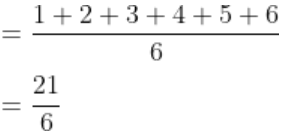= 3.5

3. Find the mean of first ten odd natural number.

Solution:

First ten odd natural numbers are 1, 3, 5, 7, 9, 11, 13, 15, 17, 19

Mean of first ten odd numbers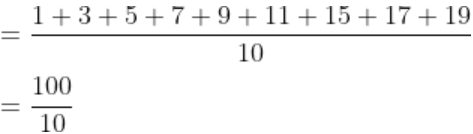4. Find the mean of all factors of 10.

Solution:

All factors of 10 are 1, 2, 5, 10

Mean of all factors of 10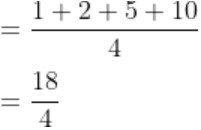= 4.5

5. Find the mean of x + 3, x + 5, x + 7, x + 9 and x + 11.

Solution:

Values given are x + 3, x + 5, x + 7, x + 9 and x + 11

Mean of the values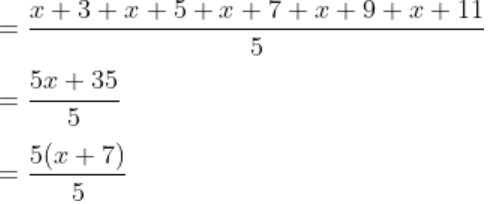= x + 7

6. If the different values of variable x are 9.8, 5.4, 3.7, 1.7, 1.8, 2.6, 2.8, 8.6, 10.5 and 11.1; find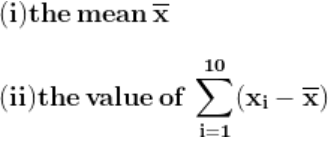Solution:

(i) Numbers given are 9.8, 5.4, 3.7, 1.7, 1.8, 2.6, 2.8, 8.6, 10.5 and 11.1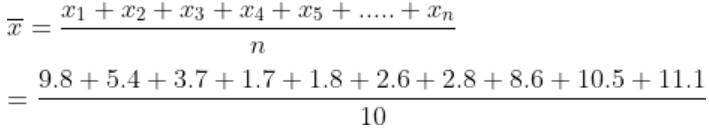= 5.8

(ii)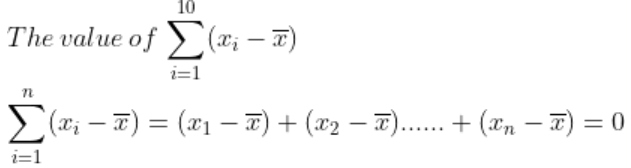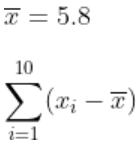= (9.8 – 5.8) + (5.4 – 5.8) + (3.7 – 5.8) + (1.7 – 5.8) + (1.8 – 5.8) + (2.6 – 5.8) + (8.6 – 5.8) + (10.5 – 5.8) + (11.1 – 5.8)

= 4 – 4 – 2.1 – 4.1 – 4 – 3.2 – 3 + 2.8 + 4.7 + 5.3

= 0

7. The mean of 15 observations is 32. Find the resulting mean, if each observation is:

(i) Increased by 3

(ii) Decreased by 7

(iii) Multiplied by 2

(iv) Divided by 0.5

(v) Increased by 60%

(vi) Decreased by 20%

Solution:

It is given that

Mean of 15 observations is 32

(i) Resulting mean if each observation is increased by 3 = 32 + 3 = 35

(ii) Resulting mean if each observation is decreased by 7 = 32 – 7 = 25

(iii) Resulting mean if each observation is multiplied by 2 = 32 × 2 = 64

(iv) Resulting mean if each observation is divided by 0.5 = 32/0.5 = 64

(v) Resulting mean if each observation is increased by 60% = 32 + 60/100 × 32

= 32 + 19.2

= 51.2

(vi) Resulting mean if each observation is decreased by 20% = 32 – 20/100 × 32

= 32 – 6.4

= 25.6

8. The mean of 5 numbers is 18. If one number is excluded, the mean of remaining number becomes 16. Find the excluded number.

Solution:

It is given that

Mean of 5 numbers is 18

Total sum of 5 numbers = 18 × 5 = 90

Excluding an observation, the mean of the remaining 4 number becomes 16 = 16 × 4 = 64

Sum of remaining 4 observations = Total of 5 observations – Total of 4 observations

= 90 – 64

= 26

9. If the mean of observations x, x + 2, x + 4, x + 6 and x + 8 is 11, find:

(i) The value of x;

(ii) The mean of first three observations.

Solution:

(i) It is given that

Mean of observations x, x + 2, x + 4, x + 6 and x + 8 is 11

We know that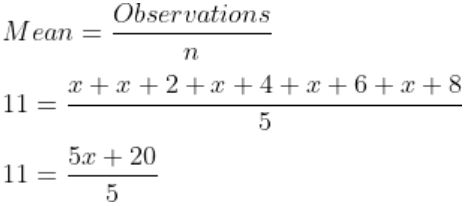By taking out 5 as common

11 = [5 (x + 4)]/5

11 = x + 4

By transposing we get

x = 11 – 4

x = 7

(ii) Mean of first three observations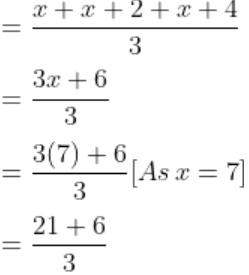= 9

10. The mean of 100 observations is 40. It is found that an observation 53 was misread as 83. Find the correct mean.

Solution:

It is given that

Mean of 100 observations is 40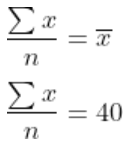x = 40 × 100 = 4000

Here the incorrect value of x = 4000

So the correct value of x = Incorrect value of x – Incorrect observation + Correct observation

Substituting the values

= 4000 – 83 + 53

= 3970

We know that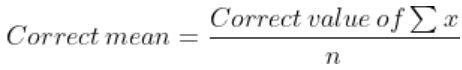= 3970/100

= 39.7

Exercise 19B page: 241

1. Find the median of:

(i) 25, 16, 26, 16, 32, 31, 19, 28 and 35

(ii) 241, 243, 347, 350, 327, 299, 261, 292, 271, 258 and 257

(iii) 63, 17, 50, 9, 25, 43, 21, 50, 14 and 34

(iv) 233, 173, 189, 208, 194, 194, 185, 200 and 220.

Solution:

(i) Arrange the numbers in ascending order

16, 16, 19, 25, 26, 28, 31, 32, 35

As n = 9 (odd)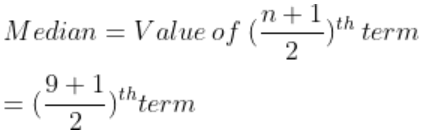= 5th term

= 26

Hence, the median is 26.

(ii) Arrange the numbers in ascending order

241, 243, 257, 258, 261, 271, 292, 299, 327, 347, 350

As n = 11 (odd)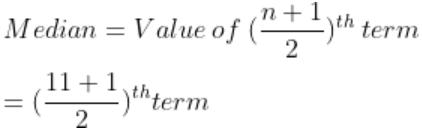= 6th term

= 271

Hence, the median is 271.

(iii) Arrange the numbers in ascending order

9, 14, 17, 21, 25, 34, 43, 50, 50, 63

As n = 10 (even)

Median = ½ [value of (n/2)th term + value of (n/2 + 1)th term]

= ½ [value of (10/2)th term + value of (10/2 + 1)th term]

= ½ [25 + 34]

= ½ 

= 29.5

Hence, the median is 29.5.

(iv) Arrange the numbers in ascending order

173, 185, 189, 194, 194, 200, 204, 208, 220, 223

As n = 10 (even)

Median = ½ [value of (n/2)th term + value of (n/2 + 1)th term]

= ½ [value of (10/2)th term + value of (10/2 + 1)th term]

= ½ [200 + 194]

= ½ 

= 197

Hence, the median is 197.

2. The following data have been arranged in ascending order. If their median is 63, find the value of x.

34, 37, 53, 55, x, x + 2, 77, 83, 89 and 100.

Solution:

Numbers given are 34, 37, 53, 55, x, x + 2, 77, 83, 89 and 100

As n = 10 (even)

Median = ½ [value of (n/2)th term + value of (n/2 + 1)th term]

= ½ [value of (10/2)th term + value of (10/2 + 1)th term]

= ½ [value of 5th term + value of (5 + 1)th term]

= ½ [value of 5th term + value of 6th term]

Substituting the values

63 = ½ [x + x + 2]

[2 + 2x]/2 = 63

Taking 2 as common

x + 1 = 63

x = 62

3. In 10 numbers, arranged in increasing order, the 7th number is increased by 8, how much will the median be changed?

Solution:

We know that for any given set of data, the median is the value of its middle term

Total observations n = 10 (even)

Median = ½ [value of (n/2)th term + value of (n/2 + 1)th term]

= ½ [value of (10/2)th term + value of (10/2 + 1)th term]

= ½ [value of 5th term + value of 6th term]

Therefore, if the 7th number is diminished by 8, there will be no change in the median value.

4. Out of 10 students, who appeared in a test, three secured less than 30 marks and 3 secured more than 75 marks. The marks secured by the remaining 4 students are 35, 48, 66 and 40. Find the median score of the whole group.

Solution:

Total observations n = 10 (even)

Median = = ½ [value of (10/2)th term + value of (10/2 + 1)th term]

= ½ [value of 5th term + value of 6th term]

Substituting the values

Median = ½ [40 + 48]

= 88/2

= 44

Hence, the median score of the whole group is 44.

5. The median of observations 10, 11, 13, 17, x + 5, 20, 22, 24 and 53 (arranged in ascending order) is 18; find the value of x.

Solution:

Total observations n = 9 (odd)

As n is odd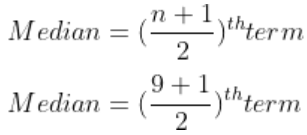= 5th term

= x + 5

It is given that, Median = 18

x + 5 = 18

x = 13

Exercise 19C

1. Find the mean of 8, 12, 16, 22, 10 and 4. Find the resulting mean, if each of the observations, given above be:

(i) Multiplied by 3.

(ii) Divided by 2.

(iii) Multiplied by 3 and then divided by 2.

(iv) Increased by 25%.

(v) Decreased by 40%.

Solution:

Mean of the given data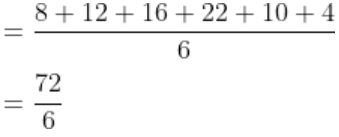= 12

(i) Multiplied by 3

If x̄ is the mean of n number of observations x1, x2, x3, ……. xn,

Mean of ax1, ax2, ax3, ……. ,axn is ax̄

When each of the given data is multiplied by 3, then mean is also multiplied by 3

Mean of the original data = 12

Therefore, the new mean = 12 × 3 = 36

(ii) Divided by 2

If x̄ is the mean of n number of observations x1, x2, x3, ……. xn,

Mean of x1/a, x2/a, x3/a, …… xn/a is x̄/a

When each of the given data is divided by 2, the mean is also divided by 2.

Mean of the original data = 12

Therefore, the new mean = 12/2 = 6

(iii) Multiplied by 3 and then divided by 2

If x̄ is the mean of n number of observations x1, x2, x3, ……. xn,

Mean of a/b x1, a/b x2, a/b x3, ….. a/b xn is a/b x̄

When each of the given data is multiplied by 3/2, the mean is also multiplied by 3/2.

Mean of original data = 12

Therefore, the new mean = 3/2 × 12 = 36/2 = 18

(iv) Increased by 25%

We know that

New mean = Original mean + 25% of original mean

New mean = 12 + 25% of 12

New mean = 12 + 25/100 × 12

So we get

New mean = 12 + ¼ × 12

New mean = 12 + 3

New mean = 15

(v) Decreased by 40%

We know that

New mean = 12 – 40% of 12

New mean = 12 – 40/100 × 12

So we get

New mean = 12 – 2/5 × 12

New mean = 12 – 0.4 × 12

New mean = 12 – 4.8

New mean = 7.2

2. The mean of 18, 24, 15, 2x + 1 and 12 is 21. Find the value of x.

Solution:

We know that

Mean of given data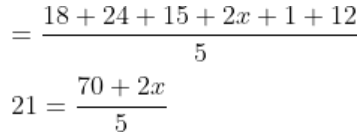By cross multiplication

5 × 21 = 70 + 2x

105 = 70 + 2x

On further calculation

2x = 105 – 70

2x = 35

x = 35/2 = 17.5

3. The mean of 6 numbers is 42. If one number is excluded, the mean of remaining number is 45. Find the excluded number.

Solution:

If x̄ is the mean of n number of observations x1, x2, x3, ……. xn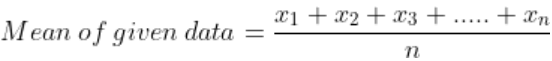It is given that mean of 6 numbers is 42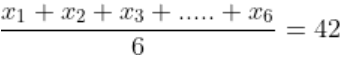x1 + x2 + x3 + …… +x6 = 42 × 6

x1 + x2 + x3 + x4 + x5 = 252 – x6 …… (1)

It is also given that the mean of 5 numbers is 45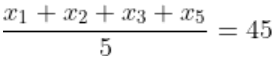x1 + x2 + x3 + x4 + x5 = 45 × 5

x1 + x2 + x3 + x4 + x5 = 225 …… (2)

From equating both the equations

252 – x6 = 225

x6 = 252 – 225 = 27

4. The mean of 10 numbers is 24. If one more number is included, the new mean is 25. Find the included number.

Solution:

If x̄ is the mean of n number of observations x1, x2, x3, ……. xnIt is given that the mean of 10 numbers is 24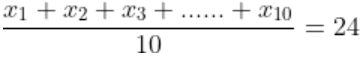x1 + x2 + x3 + …… +x10 = 24 × 10

x1 + x2 + x3 + x4 + …… +x10 = 240

x1 + x2 + x3 + x4 + …… +x10 = 240 + x11 …… (1)

It is also given that the mean of 11 numbers is 25.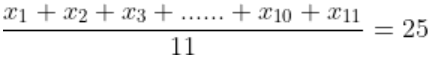x1 + x2 + x3 + x4 + …… +x10 +x11 = 11 × 25

x1 + x2 + x3 + x4 + …… +x10 +x11 = 275 ….. (2)

From equating both the equations

240 + x11 = 275

x11 = 275 – 240 = 35

5. The following observations have been arranged in ascending order. If the median of the data is 78, find the value of x.

44, 47, 63, 65, x + 13, 87, 93, 99, 110.

Solution:

The data given is

44, 47, 63, 65, x + 13, 87, 93, 99, 110

Total number of observations (n) = 9 which is odd= 5th term

= x + 13

It is given that the median is 78

x + 13 = 78

x = 78 – 13 = 65

## Selina Solutions for Class 9 Maths Chapter 19- Mean and Median

The Chapter 19, Mean and Median, contains 3 exercises and the Solutions given here contains the answers for all the questions present in these exercises. Let us have a look at some of the topics that are being discussed in this chapter.

19.1 Mean of Ungrouped data

19.2 Properties of mean

19.3 Median

## Selina Solutions for Class 9 Maths Chapter 19- Mean and Median

In chapter 19 of Class 9, the students are taught about a process in which the mean and median of a given data is calculated. Mean is the average of a given data. Study the Chapter 19 of Selina textbook to understand more about Mean and Median. Learn the Selina Solutions for Class 9 effectively to come out with flying colours in the examinations.